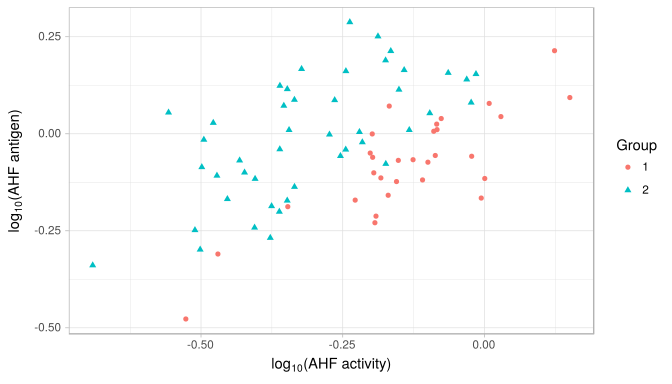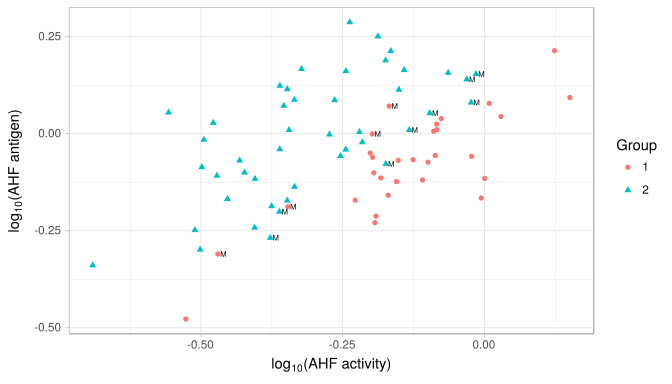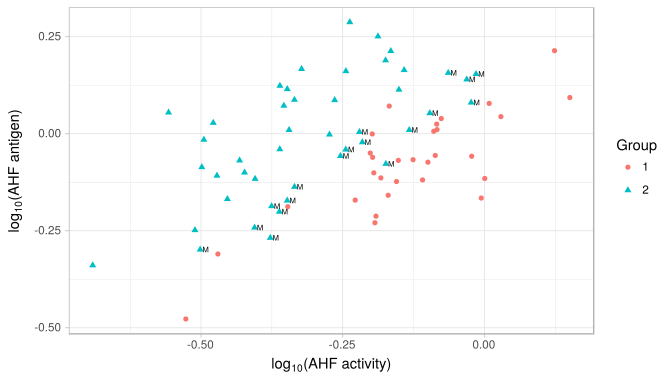$$\newcommand{\bm}{\boldsymbol{\mathbf{#1}}}$$

## 11.1

1. $$~~~~\hat{y} = (\bar{x_1} - \bar{x_2})^{\prime}S^{-1}_{pooled}x = \hat{a}^{\prime}x$$
$$~~~~~~~S^{-1}_{pooled} = \begin{bmatrix} 2 & -1 \\ -1 & 1 \end{bmatrix}$$
$$~~~~~~~\begin{bmatrix} -2 & -2 \end{bmatrix}\begin{bmatrix} 2 & -1 \\ -1 & 1 \end{bmatrix}x = -2x_1$$
1. $$~~~~\hat{m} = \frac{1}{2}(\hat{y_1}+\hat{y_2}) = -8$$
$$~~~~~~-2(2) = -4 > -8 \Rightarrow assign~to~\pi_1$$

## 11.4

1. $$~~~~(\frac{c(1\mid 2)}{c(2\mid 1)})(\frac{p_2}{p_1}) = 0.5$$
$$~~~~~~~\Rightarrow assign~to~\pi_1~if~\frac{f_1(x)}{f_2(x)}\geq0.5,~otherwise~assign~to~\pi_2$$
1. $$~~~~\frac{f_1(x)}{f_2(x)} = \frac{0.3}{0.5} = 0.6 > 0.5\Rightarrow assign~to~\pi_1$$

## 11.19

1. $$~~~~\hat{y_0} = \hat{a}^{\prime}x_0 = -0.33x_1+0.67x_2$$
$$~~~~~~~where~\hat{m} = 4.5$$
$$~~~~~~~assign~to~\pi_1~if~-0.33x_1+0.67x_2-4.5\geq 0,~otherwise~assign~to~\pi_2$$
$$\pi_1$$ $$\pi_2$$
obs. $$−0.33x_1+0.67x_2−4.5$$ class. obs. $$−0.33x_1+0.67x_2−4.5$$ class.
1 2.83 $$\pi_1$$ 1 -1.5 $$\pi_2$$
2 0.83 $$\pi_1$$ 2 0.5 $$\pi_1$$
3 -0.17 $$\pi_2$$ 3 -2.5 $$\pi_2$$
1. $$~~~~D^2_i(x) = (x - \bar{x_i})^{\prime}S^{-1}_{pooled}(x - \bar{x_i})$$
$$\pi_1$$ $$\pi_2$$
obs. $$D^2_1$$ $$D^2_2$$ class. obs. $$D^2_1$$
1 1.33 7 $$\pi_1$$ 1 4.33
2 1.33 3 $$\pi_1$$ 2 0.33
3 1.33 1 $$\pi_2$$ 3 6.33

## 11.32

### (a) Bivariate plotThe bivariate plot doesn’t seem to fit well into a bivariate normal distribution.

### (b) linear disciminant function

$\begin{equation} \begin{split} \hat{y} &= (\bar{\bm{x}}_1 - \bar{\bm{x}}_2)^T S_{pool}^{-1} ~ \bm{x}_0 \\ &= \bm{a}^T \bm{x}_0 \\ &= \begin{pmatrix} 19.319 & -17.124 \end{pmatrix} \bm{x}_0 \end{split} \tag{1} \end{equation}$

Then allocate $$\bm{x}_0$$ to $$\pi_1$$ if:

$\begin{equation} \begin{split} \hat{y} &\geq \frac{1}{2} (\bar{\bm{x}}_1 - \bar{\bm{x}}_2)^T S_{pool}^{-1} ~ (\bar{\bm{x}}_1 + \bar{\bm{x}}_2) \\ &= \frac{1}{2} \bm{a}^T (\bar{\bm{x}}_1 + \bar{\bm{x}}_2) = -3.559 \end{split} \tag{2} \end{equation}$

where, $$\bar{\bm{x}}_1 =\begin{pmatrix} -0.135 \\ -0.078 \end{pmatrix}, \bar{\bm{x}}_2 = \begin{pmatrix} -0.308 \\ -0.006 \end{pmatrix}$$

The linear discriminant function is:

$\begin{equation} 19.319 x_{0,1} -17.124 x_{0,1} + 3.559 \tag{3} \end{equation}$

The confusion matrix constructed with the holdout procedure is

Group 1 Group 2
1 26 4
2 8 37

, and the estimated error rate is 0.16.

The misclassified observations are No. 3, 5, 7, 17, 32, 35, 58, 62, 63, 64, 67, 69, labeled “M” in the plot below.### (c)

By eq. (1) and eq. (2), $$\hat{y}_0 = 2.614 \geq -3.559$$. Hence, it is allocated to Group 1.

### (d)

Classification rule based on posterior probabilities is equivalent to classification rule based on minimizing TPM.

Since the prior probabilities are assumed to be equal, the posterior probabilities are calculated as:

$\begin{equation} \begin{split} p(\pi_1|\bm{x}_0) &= \frac{ f_1(\bm{x}_0)}{f_1(\bm{x}_0) + f_2(\bm{x}_0)} = 0.9608785 \\ p(\pi_2|\bm{x}_0) &= 1 - p(\pi_1|\bm{x}_0) = 0.0389789 \end{split} \tag{4} \end{equation}$

, where the densities $$f_1(\bm{x}_0)$$ and $$f_2(\bm{x}_0)$$ are assumed to be normal and are estimated using $$\bar{\bm{x}}_1, \bar{\bm{x}}_2, \bm{S}_1, \bm{S}_2$$.

By $$p(\pi_1|\bm{x}_0) > p(\pi_2|\bm{x}_0)$$, $$\bm{x}_0$$ is classified as Group 1.

### (e)

By eq. (1), eq. (2), and c, the linear discriminant score is calculated as $$\hat{y}_0 - \frac{1}{2} (\bar{\bm{x}}_1 - \bar{\bm{x}}_2)^T S_{pool}^{-1} ~ (\bar{\bm{x}}_1 + \bar{\bm{x}}_2) = 6.173$$.

### (f)

Assume $$p_1 = 0.75$$ and $$p_2 = 0.25$$, then allocate $$\bm{x}_0$$ to $$\pi_1$$ if:

$\begin{equation} \begin{split} \hat{y} &\geq \frac{1}{2} (\bar{\bm{x}}_1 - \bar{\bm{x}}_2)^T S_{pool}^{-1} ~ (\bar{\bm{x}}_1 + \bar{\bm{x}}_2) + ln (\frac{c(1|2) p_2}{c(2|1) p_1}) \\ &= \frac{1}{2} \bm{a}^T (\bar{\bm{x}}_1 + \bar{\bm{x}}_2) + ln (\frac{0.25}{0.75}) = -4.658 \end{split} \tag{5} \end{equation}$

where, $$\bar{\bm{x}}_1 =\begin{pmatrix} -0.135 \\ -0.078 \end{pmatrix}, \bar{\bm{x}}_2 = \begin{pmatrix} -0.308 \\ -0.006 \end{pmatrix}$$

The linear discriminant function is:

$\begin{equation} 19.319 x_{0,1} -17.124 x_{0,1} + 4.658 \tag{6} \end{equation}$

The confusion matrix constructed with the holdout procedure is

Group 1 Group 2
1 30 0
2 18 27

, and the estimated error rate is 0.24.

The misclassified observations are No. 32, 34, 35, 39, 47, 51, 54, 55, 57, 58, 60, 61, 62, 63, 64, 67, 69, 73, labeled “M” in the plot below.### (g)

By eq. (1) and eq. (5), $$\hat{y}_0 - [ \frac{1}{2} \bm{a}^T (\bar{\bm{x}}_1 + \bar{\bm{x}}_2) + ln (\frac{0.25}{0.75}) ] = 7.272 > 0$$. Hence, it is allocated to Group 1.

### (h)

Classification rule based on posterior probabilities is equivalent to classification rule based on minimizing TPM.

The posterior probabilities are calculated as:

$\begin{equation} \begin{split} p(\pi_1|\bm{x}_0) &= \frac{ p_1 f_1(\bm{x}_0)}{p_1 f_1(\bm{x}_0) + p_2 f_2(\bm{x}_0)} = 0.9876759 \\ p(\pi_2|\bm{x}_0) &= 1 - p(\pi_1|\bm{x}_0) = 0.0133553 \end{split} \tag{7} \end{equation}$

, where the densities $$f_1(\bm{x}_0)$$ and $$f_2(\bm{x}_0)$$ are assumed to be normal and are estimated using $$\bar{\bm{x}}_1, \bar{\bm{x}}_2, \bm{S}_1, \bm{S}_2$$.

By $$p(\pi_1|\bm{x}_0) > p(\pi_2|\bm{x}_0)$$, $$\bm{x}_0$$ is classified as Group 1.

### (i)

By eq. (1), eq. (5), and g, the linear discriminant score is calculated as $$\hat{y}_0 - [ \frac{1}{2} \bm{a}^T (\bar{\bm{x}}_1 + \bar{\bm{x}}_2) + ln (\frac{0.25}{0.75}) ] = 7.271934$$.